Next: Exercises Up: Newtonian mechanics Previous: Simple harmonic motion

# Two-body problem

An isolated dynamical system consisting of two freely moving point objects exerting forces on one another is conventionally termed a two-body problem. Suppose that the first object is of mass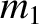and is located at position vector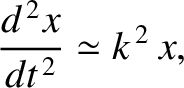. Likewise, the second object is of mass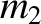and is located at position vector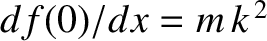. Let the first object exert a force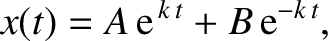on the second. By Newton's third law, the second object exerts an equal and opposite force,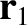, on the first. Suppose that there are no other forces in the problem. The equations of motion of our two objects are thus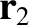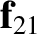(2.71) and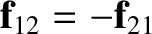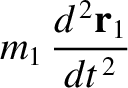(2.72)

where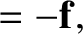.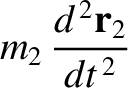Now, the center of mass of our system is located at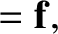(2.73)

Hence, we can write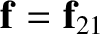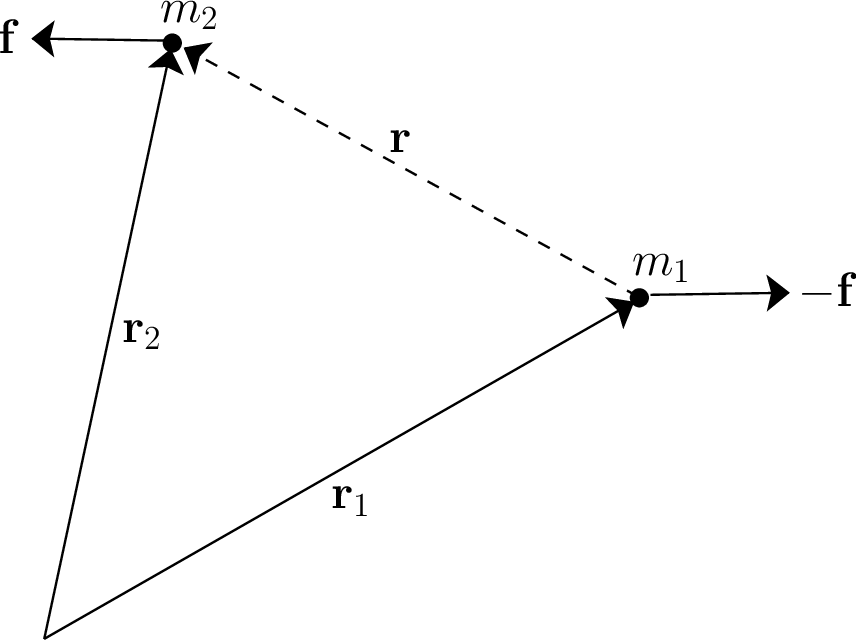(2.74) and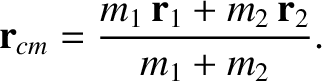(2.75)

where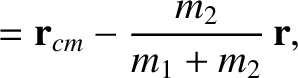. (See Figure 2.7.) Substituting the preceding two equations into Equations (2.71) and (2.72), and making use of the fact that the center of mass of an isolated system does not accelerate (see Section 2.5), we find that both equations yield(2.76)

where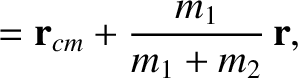(2.77)

is called the reduced mass. Hence, we have effectively converted our original two-body problem into an equivalent one-body problem. In the equivalent problem, the force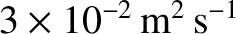is the same as that acting on both objects in the original problem (except for a minus sign); however, the mass,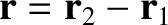, is different, and is less than either ofor(which is why it is called the reduced'' mass). We conclude that the dynamics of an isolated system consisting of two interacting point objects can always be reduced to that of an equivalent system consisting of a single point object moving in a fixed force field.Next: Exercises Up: Newtonian mechanics Previous: Simple harmonic motion
Richard Fitzpatrick 2016-03-31